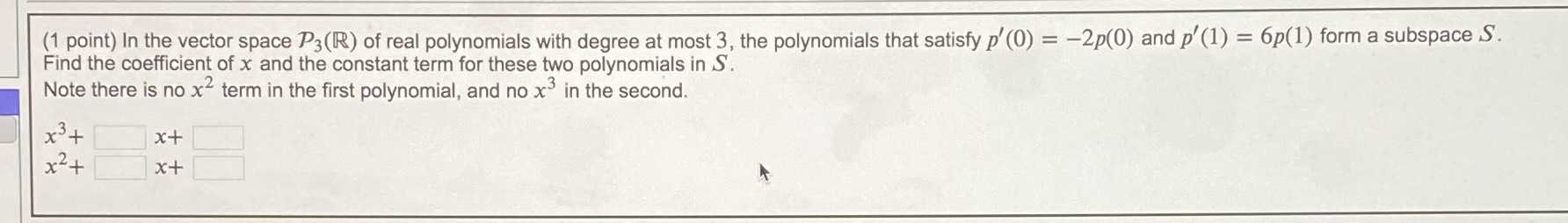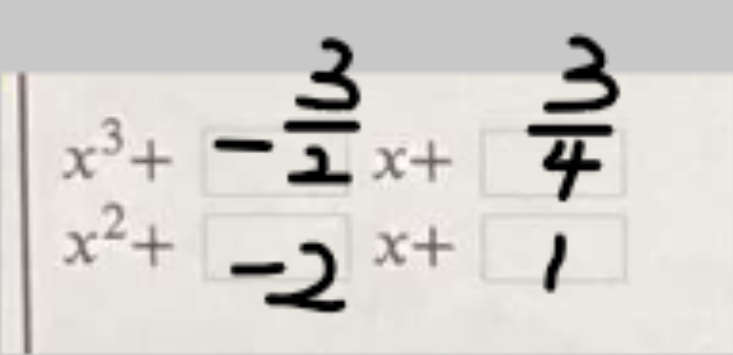### ¿Todavía tienes preguntas de matemáticas?

Pregunte a nuestros tutores expertos
Algebra
Pregunta(1 point) In the vector space $$P _ { 3 } ( R )$$ of real polynomials with degree at most $$3 ,$$ the polynomials that satisfy $$p ^ { \prime } ( 0 ) = - 2 p ( 0 )$$ and $$p ^ { \prime } ( 1 ) = 6 p ( 1 )$$ form a subspace $$S$$ . Find the coefficient of $$x$$ and the constalt term for these two polynomials in $$S$$ . Note there is no $$x ^ { 2 }$$ term in the first polynomial, and no $$x ^ { 3 }$$ in the second.